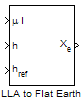Documentation

# LLA to Flat Earth

Estimate flat Earth position from geodetic latitude, longitude, and altitude

## Library

Utilities/Axes Transformations## Description

The LLA to Flat Earth block converts a geodetic latitude $\left(\overline{\mu }\right)$, longitude $\left(\overline{\iota }\right)$, and altitude (h) into a 3-by-1 vector of Flat Earth position$\left(\overline{p}\right)$. Latitude and longitude values can be any value. However, latitude values of +90 and -90 may return unexpected values because of singularity at the poles. The flat Earth coordinate system assumes the z-axis is downward positive. The estimation begins by finding the small changes in latitude and longitude from the output latitude and longitude minus the initial latitude and longitude.

`$\begin{array}{l}d\mu =\mu -{\mu }_{0}\\ d\iota =\iota -{\iota }_{0}\end{array}$`

To convert geodetic latitude and longitude to the North and East coordinates, the estimation uses the radius of curvature in the prime vertical (RN) and the radius of curvature in the meridian (RM). RN and RM are defined by the following relationships:

`$\begin{array}{l}{R}_{N}=\frac{R}{\sqrt{1-\left(2f-{f}^{2}\right){\mathrm{sin}}^{2}{\mu }_{0}}}\\ {R}_{M}={R}_{N}\frac{1-\left(2f-{f}^{2}\right)}{1-\left(2f-{f}^{2}\right){\mathrm{sin}}^{2}{\mu }_{0}}\end{array}$`

where (R) is the equatorial radius of the planet and $f$ is the flattening of the planet.

Small changes in the North (dN) and East (dE) positions are approximated from small changes in the North and East positions by

`$\begin{array}{l}dN=\frac{d\mu }{\text{atan}\left(\frac{1}{{R}_{M}}\right)}\\ dE=\frac{d\iota }{\text{atan}\left(\frac{1}{{R}_{N}\mathrm{cos}{\mu }_{0}}\right)}\end{array}$`

With the conversion of the North and East coordinates to the flat Earth x and y coordinates, the transformation has the form of

`$\left[\begin{array}{c}{p}_{x}\\ {p}_{y}\end{array}\right]=\left[\begin{array}{cc}\mathrm{cos}\psi & \mathrm{sin}\psi \\ -\mathrm{sin}\psi & \mathrm{cos}\psi \end{array}\right]\left[\begin{array}{c}N\\ E\end{array}\right]$`

where

`$\left(\psi \right)$`

is the angle in degrees clockwise between the x-axis and north.

The flat Earth z-axis value is the negative altitude minus the reference height (href).

`${p}_{z}=-h-{h}_{ref}$`

## Parameters

Units

Specifies the parameter and output units:

Units

Position

Altitude

`Metric (MKS)`

Meters

Meters

Meters

`English`

Feet

Feet

Feet

This option is available only when Planet model is set to `Earth (WGS84)`.

Planet model

Specifies the planet model to use: `Custom` or ```Earth (WGS84)```.

Flattening

Specifies the flattening of the planet. This option is available only with Planet model Custom.

Specifies the radius of the planet at its equator. The units of the equatorial radius parameter should be the same as the units for flat Earth position. This option is available only with Planet model Custom.

Initial geodetic latitude and longitude

Specifies the reference location, in degrees of latitude and longitude, for the origin of the estimation and the origin of the flat Earth coordinate system.

Direction of flat Earth x-axis

Specifies angle for converting flat Earth x and y coordinates to North and East coordinates.

## Inputs and Outputs

InputDimension TypeDescription

First

2-by-1 vectorContains the geodetic latitude and longitude, in degrees.

Second

ScalarContains the altitude above the input reference altitude, in same units as flat Earth position.

Third

ScalarContains the reference height from the surface of the Earth to the flat Earth frame, in same units as flat Earth position. The reference height is estimated with regard to Earth frame.
OutputDimension TypeDescription

First

3-by-1 vectorContains the position in flat Earth frame.

## Assumptions and Limitations

This estimation method assumes the flight path and bank angle are zero.

This estimation method assumes the flat Earth z-axis is normal to the Earth at the initial geodetic latitude and longitude only. This method has higher accuracy over small distances from the initial geodetic latitude and longitude, and nearer to the equator. The longitude has higher accuracy with smaller variations in latitude. Additionally, longitude is singular at the poles.

## References

Etkin, B. Dynamics of Atmospheric Flight New York: John Wiley & Sons, 1972.

Stevens, B. L., and F. L. Lewis. Aircraft Control and Simulation, 2nd ed. New York: John Wiley & Sons, 2003.

#### Introduced in R2011a

##### SupportGet trial now